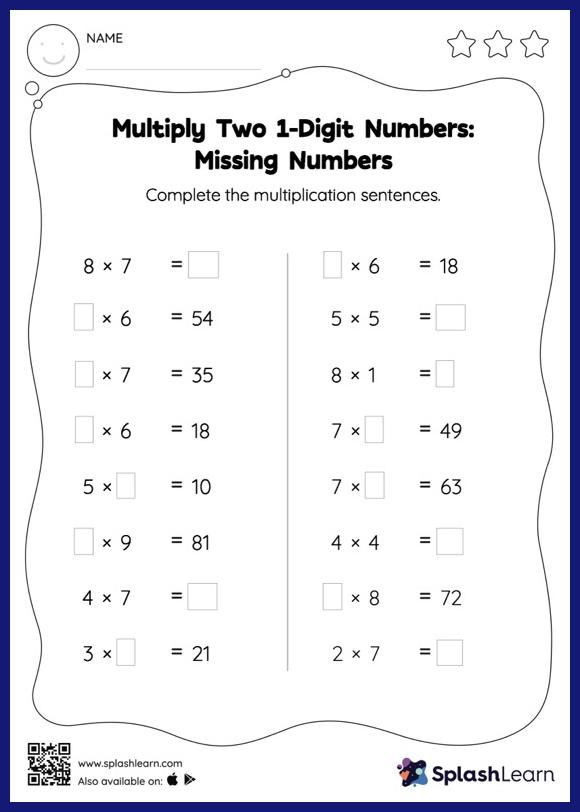# Multiply Two 1-Digit Numbers: Missing Numbers Worksheet

Home > Multiply Two 1-Digit Numbers: Missing NumbersChallenge your young math wizards to solve this multiply two 1-digit numbers worksheet. Students multiply one digit at a time when multiplying a multi-digit number by a one-digit number. They use their knowledge of basic multiplication facts to find the missing number in multiply two 1-digit numbers worksheet. In each problem, the numbers are laid out in the horizontal format. Students should try to use different strategies involving composing and decomposing numbers to solve these problems. This will help them develop flexibility and fluency.